# Test: Geometry- 2

## 15 Questions MCQ Test IBPS PO Prelims- Study Material, Online Tests, Previous Year | Test: Geometry- 2

Description
Attempt Test: Geometry- 2 | 15 questions in 15 minutes | Mock test for UPSC preparation | Free important questions MCQ to study IBPS PO Prelims- Study Material, Online Tests, Previous Year for UPSC Exam | Download free PDF with solutions
QUESTION: 1

### A cyclic quadrilateral is such that two of its adjacent angles are divisible by 6 and 10 respectively. One of the remaining angles will necessarily be divisible by:

Solution:

The two adjacent angles can be 30° and 60°, therefore others angle can be 150° and 120°

QUESTION: 2

### The volume of spheres are proportional to the cubes of their radii. Two spheres of the same material weigh 3.6 kg and 2.7 kg and the radius of the smaller one is 2 cm. If the two were melted down and formed into a single sphere, what would be its radius?

Solution: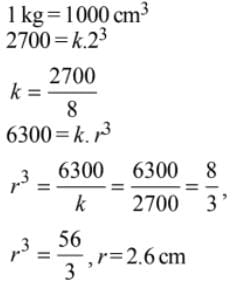QUESTION: 3

### Read the passage below and solve the questions based on it fix) is the area of a square where, Jr is the side of a square. g(x) is the perimeter of square where, Jr is the side of a square. h(xt y) is the area of a rectangle where* is the length and y is the breadth. i(xt y) is the perimeter of a rectangle where Jt is the length and y is the breadth.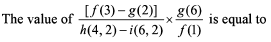Solution: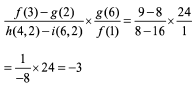QUESTION: 4

Read the passage below and solve the questions based on it.
A 10 m long piece of wire is cut into two pieces, one of which is bent into a circle and the other into the square enclosing it.

Q.

The ratio of the radius of the circle to the perimeter of the square is

Solution: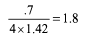QUESTION: 5

A square is inscribed in a semi circle of radius 10 cm. What is the area of the inscribed square? (Given that the side of the square is along the diameter of the semicircle.)

Solution: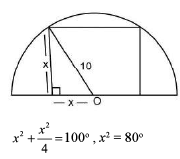QUESTION: 6

Two circles of an equal radii are drawn, without any overlap, in a semicircle of radius 2 cm. If these are the largest possible circles that the semicircle can accommodate, what is the radius (in cm) of each of the circles?

Solution: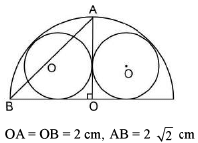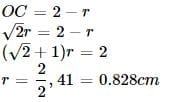QUESTION: 7

PQRS is a Trapezzium, in which PQ is Parralel to RS, and PQ = 3 (RS) . The diagnol of the Trapezzium intersect each other at X, then the ratio of,  ar ( ∆ PXQ)  : ar ( ∆ RXS)  is?

Solution: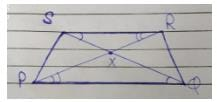In ∆ PXQ and ∆ RXS

=> angle P = angle R

angle Q  =  angle S

:- ∆ PXQ  ~ ∆ RXS  ( AA similarity rule)

ar ( ∆ PXQ) /  ar ( ∆ RXS) = ( PQ / RS) ^ 2

=  ( 3 / 1 ) ^ 2

=     9 / 1

Therefore,  ar ( ∆ PXQ) :  ar          ( ∆ RXS)

=   9:1

QUESTION: 8

Let ABCDEF be a regular hexagon. What is the ratio of the area of the triangle ACE to that of the hexagon ABCDEF?

Solution: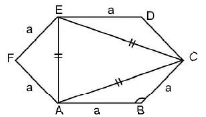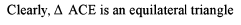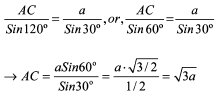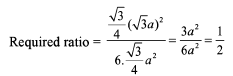QUESTION: 9

A pond 100 m in diameter is surrounded by a circular grass walk-way 2 m wide. How many square metres of grass is the on the walk-way?

Solution: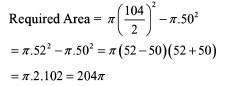QUESTION: 10

The dimensions of a rectangular box are in the ratio of 1:2:4 and the difference between the costs of covering it with the cloth and a sheet at the rate of Rs 20 and Rs 20.5 per sq m respectively is Rs 126. Find the dimensions of the box.

Solution: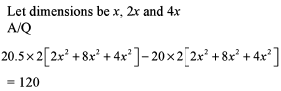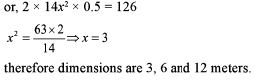QUESTION: 11

The ratio of the area of a square inscribed in a semicircle to that of the area of a square inscribed in the circle of the same radius is

Solution: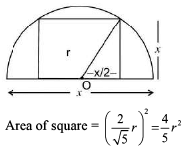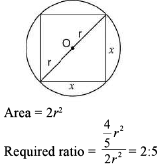QUESTION: 12

The ratio of the area of a square to that of the square drawn on the its the diagonal is

Solution: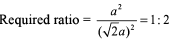QUESTION: 13

What is the area of the triangle in which two of its medians 9 cm and 12 cm long intersect at the right angles?

Solution: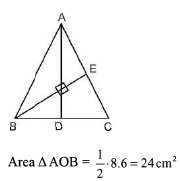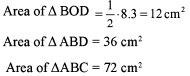QUESTION: 14

Four horses are tethered at four comers of a square plot of side 14 m so that the adjacent horses can just reach one another. There is a small circular pond of area 20 m2 at the centre. Find the ungrazed area.

Solution:

Total area of plot = 14 * 14 = 196m2
Horses can graze in quarter circle of radius = 7m
Grazed area = 4 * (pie r2)/4 = 154 m2
Area of plot when horses cannot reach = (196 - 154) = 42m2
Ungrazed area = 42 - 20 = 22m2

QUESTION: 15

Two sides of a triangle are 4 and 5. Then, for the area of the triangle, which one of the following bounds is the sharpest?

Solution:

Let AB = 4 and BC = 5 and AB is perpendicular to BC

then Area = 1/2 AB . AC = 1/2 . 4.5 = 10Use Code STAYHOME200 and get INR 200 additional OFF Use Coupon Code